# Binary heap

A binary heap is a complete binary tree in which nodes are labelled with elements from a totally ordered set and each node's label is greater than the labels of its children, if any. (We call this variation the max heap, because the maximum element is at the root; the min heap is defined analogously.) It is used to implement the priority queue abstract data type.

## Details

The unifying principle of binary heap operations is that they must never violate the completeness property, but may temporarily violate the max-heap property.

### Finding the maximum

The maximum node in a heap is always at the top. This is obvious from the fact that every node other than the root has a parent (that is greater than or equal to it).

### Insertion

Inserting a node into an empty heap is easy; all we have to do is make it the root.

Otherwise, when a node is inserted, there is only one possible place for it; either it goes immediately to the right of the rightmost node on the deepest level, or, if the bottom level is already full, it begins a new level, taking the leftmost position there.

If this node is less than or equal to its parent, we are done already; we have a new heap that contains all the original nodes plus the new one and the max-heap property is satisfied. Otherwise we must bottom-up heapify the new tree. That is, if the node we just inserted is greater than its parent, we must swap the node with its parent, and if it is greater than its new parent, we must swap again, and so on. This process will terminate either when the node is less than or equal to its parent, or when the node reaches the root (which occurs if and only if it is the greatest element in the heap).

It is easy to see that bottom-up heapification results in the restoration of the max-heap property. The proof is left as an exercise to the reader.

### Deletion

Only the greatest element in a max heap can be deleted. Of course, we can't delete it while it is still at the root of the heap (unless the heap contains only a single element). Instead, we will remove the rightmost node on the bottom level, because we want to maintain the completeness property of the heap. Thus, we will start by swapping the label of the root node with the label of the rightmost node on the bottom level, and then removing the latter.

If the node now at the root is greater than or equal to both of its children, then we are done. Otherwise, we must perform a top-down heapify. Since the node is less than at least one of its children, we reason that it is less than the greater of its two children. So we will swap the node with the greater of its two children; the new root will now be greater than both of its children. However, if the node previously at the root is still less than at least one of its children, we must swap it down again, and so on; this process will terminate either when the node is greater than or equal to both of its children or when it reaches the bottom of the heap (i.e., becomes a leaf), and no longer has children.

The proof that top-down heapification results in the restoration of the max-heap property is left as an exercise to the reader.

### Increasing a key

If we know where in the heap a given key (label) can be found, we can efficiently change the key's value. If we increase the key's value, we may have to perform bottom-up heapification starting at the node; if we decrease the key's value, we may have to perform top-down heapification. The former is more common, and occurs, for example, in Dijkstra's algorithm and Prim's algorithm, when we update distances. (Note that in this case we would use a min heap and decrease the key.)

### Construction

Given a set of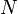$N$ labels, we can construct a heap containing$N$ nodes, each of which has one of the given labels, simply by performing$N$ insertions. However, a more efficient technique is bottom-up construction. This technique entails first constructing a complete binary tree with all$N$ labels, in any order, and then performing top-down heapification on each node, in decreasing order of depth. The proof that this results in a valid binary heap is not difficult; heapifying at a given node may be thought of as being like "adding" the node at the top.

The reason for constructing the heap in this manner will become evident when we analyze the running time of the implementations of these operations.

## Analysis

We use the fact that the height of a binary heap with$N$ nodes is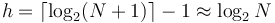$h = \lceil \log_2(N+1) \rceil - 1 \approx \log_2 N$.

Average-case analyses are difficult, as they make assumptions about the likely distribution of keys to be inserted as well as the distribution of keys that are already in the heap. The analyses given below are not rigorous.

### Finding the maximum

Simply looking at the root of the heap takes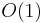$O(1)$ time.

### Insertion

A bottom-up heapification, in the worst case, uses approximately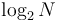$\log_2 N$ swaps (if it goes all the way up to the top of the tree), each of which is assumed to take constant time, giving$O(\log N)$. However, on average, the newly inserted element does not travel very far up the tree. In particular, assuming a uniform distribution of keys, it has a one-half chance of being greater than its parent; it has a one-half chance of being greater than its grandparent given that it is greater than its parent; it has a one-half chance of being greater than its great-grandparent given that it is greater than its parent, and so on, so that the expected number of swaps is$\frac{1}{2} + \frac{1}{4} + \frac{1}{8} + \frac{1}{16} + ... = 1$, so that in the average case insertion takes constant time,$O(1)$ (and the expected final depth of the node is$h-1$).

(A madly handwaving way to reach the same conclusion is to claim that the newly inserted node has an equal probability of ending up anywhere in the heap, so the average final depth is about$(1/2)h + (1/4)(h-1) + (1/8)(h-2) + ... \approx h-1$ since about half the nodes in a complete binary tree are on the bottom level, about a quarter are on the level above that, and so on. Obviously, this premise is false, but it gives the same result.)

### Deletion

A top-down heapification, in the worst case, uses approximately$\log_2 N$ swaps (if it goes all the way back down to the bottom of the tree), each of which is assumed to take constant time, giving$O(\log N)$ time. In the average case, the analysis is more complicated. Assume, for simplicity, uniform distribution of keys and assume that no two keys are equal. Consider the path from the root to the now vacated original position of the key that now occupies the root. As long as the key stays on this path, it will have to be swapped down further, because we know it is less than its child on the path, but as soon as the key leaves the path, it finds itself among a subtree of keys whose sizes relative to it are unknown and uniformly distributed. The expected final depth will then be$h-1$, as in the insertion analysis. So we find that the overall expected final depth is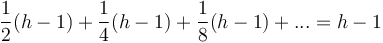$\frac{1}{2}(h-1) + \frac{1}{4}(h-1) + \frac{1}{8}(h-1) + ... = h-1$ (where the first term corresponds to the key leaving the path on the first swap, the second the second swap, and so on). Thus, on average,$h-1$ swaps occur, so the average-case time for deletion is no better asymptotically than the worst-case time.

### Increasing a key

We will not attempt an analysis of the average-case running time of this operation, as the expected amount by which a key is to be increased may vary and hence cause the expected running time to vary with it. The worst case, however, remains$O(\log N)$.

### Construction

In the average case, construction by repeated insertion takes$NO(1) = O(N)$ time. However, in the worst case, it takes$O(\log 1 + \log 2 + ... + \log N)$ time, as the heap will have one node after the first insertion, two after the second insertion, and so on; this is$O(N \log N)$.

The construction technique described above, however, has the property that a node at depth$k$ will only be swapped down up to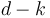$d-k$ times. Approximately half of the nodes in the heap will be on the bottom level, because such is the structure of a complete binary tree; they will therefore not be swapped down at all. Then approximately one fourth of the nodes will be on the second level from the bottom, and will be swapped down up to once; an eighth will be swapped down at most twice, and so on, giving the total number of swaps as approximately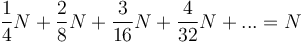$\frac{1}{4}N + \frac{2}{8}N + \frac{3}{16}N + \frac{4}{32}N + ... = N$. Thus, this procedure has a worst-case performance of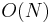$O(N)$.

## Implementation

A binary heap is almost always implemented using an array. Generally the root is placed at index one of the array, and if an element is placed at index$k$, then its left child is placed at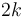$2k$ and its right at$2k+1$. Using this system, the root's left child goes at index 2, the root's right child at 3, the root's left child's left child at 4, and so on; indices from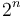$2^n$ to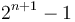$2^{n+1}-1$ are occupied by elements of depth$n$, and no space is wasted.